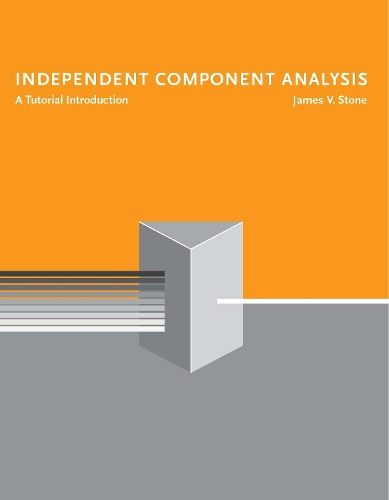•# Independent Component Analysis: A Tutorial Introduction - A Bradford Book (Paperback)

(author)
£40.00
Paperback 200 Pages / Published: 03/09/2004
• We can order this

Usually dispatched within 3 weeks

A tutorial-style introduction to a class of methods for extracting independent signals from a mixture of signals originating from different physical sources; includes MatLab computer code examples. Independent component analysis (ICA) is becoming an increasingly important tool for analyzing large data sets. In essence, ICA separates an observed set of signal mixtures into a set of statistically independent component signals, or source signals. In so doing, this powerful method can extract the relatively small amount of useful information typically found in large data sets. The applications for ICA range from speech processing, brain imaging, and electrical brain signals to telecommunications and stock predictions. In Independent Component Analysis, Jim Stone presents the essentials of ICA and related techniques (projection pursuit and complexity pursuit) in a tutorial style, using intuitive examples described in simple geometric terms. The treatment fills the need for a basic primer on ICA that can be used by readers of varying levels of mathematical sophistication, including engineers, cognitive scientists, and neuroscientists who need to know the essentials of this evolving method. An overview establishes the strategy implicit in ICA in terms of its essentially physical underpinnings and describes how ICA is based on the key observations that different physical processes generate outputs that are statistically independent of each other. The book then describes what Stone calls "the mathematical nuts and bolts" of how ICA works. Presenting only essential mathematical proofs, Stone guides the reader through an exploration of the fundamental characteristics of ICA. Topics covered include the geometry of mixing and unmixing; methods for blind source separation; and applications of ICA, including voice mixtures, EEG, fMRI, and fetal heart monitoring. The appendixes provide a vector matrix tutorial, plus basic demonstration computer code that allows the reader to see how each mathematical method described in the text translates into working Matlab computer code.

Publisher: MIT Press Ltd
ISBN: 9780262693158
Number of pages: 200
Weight: 408 g
Dimensions: 229 x 178 x 13 mm